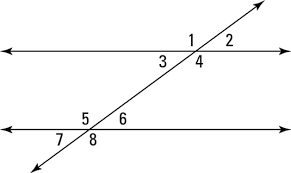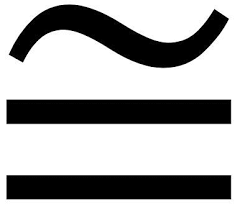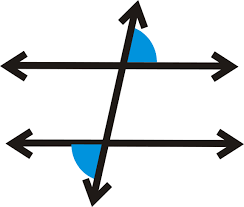Vocabulary
Vocabulary Part 2
Angle Relationships
Angle Relationships Part 2
Algebra Attack/Random Tidbits
100

Any line that intersects two parallel lines

What is a transversal

100

If two parallel lines are cut by a transversal, these angles are on the inside of the two parallel lines and on the same side of the transversal.What are Same Side Interior Angles

100

Two angles are congruent. The measure of the first angles is 42 degrees, what is the measure of the second angle

What is 42

100

If there is a pair of adjacent (side-by-side) angles formed by intersecting line, what is their relationship? What do their measures add up to be?

What is supplementary

100

What is 112% written as a decimal?

What is 1.12

200

Two angles whose measures add up to 180 degrees

What are supplementary angles

200

Two angles whose measures add up to 90 degrees

What are Complementary Angles.

200

What is the relationship between angles 4 and 5?What are Alternate Interior Angles.

200

Given that l || m, m<3=64°, then the measure of <6 is:What is 64 degrees? (Alternate Interior Angles are congruent)

200

What is the square root of 169

What is positive OR negative 13?

300

When 2 parallel lines are both intersected by a transversal, these angles occupy the same position on the top and bottom parallel lines.What are Corresponding Angles.

300

What does this symbol meanWhat is CONGRUENT

300

If the measure of angle 8 is 112, what is the measure of angle 6?What is 68.

300

Given that l || m, m<1=98°, then the measures of <8 is:What is 98 degrees (Alternate Exterior Angles are congruent)

300

Solve:

x* x?

What is x6

400

Are angles formed by two intersecting lines that are opposite of each other congruent?

Yes. They are called vertical angles

400

When 2 parallel lines are both intersected by a transversal these angles are outside of the two parallel lines and on opposite sides of the transversal.What is Alternate Exterior Angles

400

What is the relationship between angles 1 and 8?What are Alternate Exterior Angles.

400

If the measure of angle 2 is 30 degrees, what is the measure of angle 7?What is 30.

400

What is the equation for a line with a slope of 1/2 and a y intercept of -8?

What is y = 1/2 x - 8

500

When 2 parallel lines are both intersected by a transversal these angles are inside of the two parallel lines and on opposite sides of the transversal.What are Alternate Interior Angles.

500

Lines that do not intersect.

What are parallel lines.

500

If the measure of angle 1 is 37 degrees, what is the measure of angle 8? What is the angle relationship?What is 37...Alternate Exterior Angles

500

Given that l || m, m<1=98°, then the measures of <3 is:What is 82 degrees

500

Solve for the variable:

-3x - 4(4x-8) = 3(-8x-1)

What is x= -7

Click to zoom